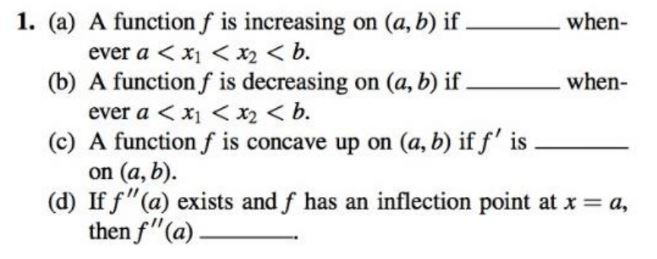# 1. (a) A function f is increasing on (a, b) if.when-ever a < x1 < x2 < b.(b) A function f is decreasing on (a, b) if .when-ever a < x1 < x2 < b.(c) A function f is concave up on (a, b) if f' ison (a, b).(d) If f"(a) exists and f has an inflection point at x = a,then f"(a)

Question
14 viewshelp_outlineImage Transcriptionclose1. (a) A function f is increasing on (a, b) if. when- ever a < x1 < x2 < b. (b) A function f is decreasing on (a, b) if . when- ever a < x1 < x2 < b. (c) A function f is concave up on (a, b) if f' is on (a, b). (d) If f"(a) exists and f has an inflection point at x = a, then f"(a) fullscreen
check_circle

Step 1

Since we are allowed to answer three subparts only and you have not mentioned the ...

### Want to see the full answer?

See Solution

#### Want to see this answer and more?

Solutions are written by subject experts who are available 24/7. Questions are typically answered within 1 hour.*

See Solution
*Response times may vary by subject and question.
Tagged in